# Context Transition when Using CALCULATE Function in Power BI

### Colin Maitland, 23 May 2019

When writing and testing DAX formulas, it is important to understand the Evaluation Context in effect for each part of the formula; i.e. Row Context and Filter Context. The purpose of the DAX CALCULATE function is to modify the Evaluation Context of an expression.

In this article, I will demonstrate Context Transition through the use of CALCULATE in two different ways; i.e. CALCULATE without the use of any Filter parameters and CALCULATE with the addition of Filter parameters that modify the Filter Context applied by Context Transition.

I have loaded a sample data set of Opportunity Products into Power BI from Dynamics 365. Some of these, all of which are related to the same Opportunity, are shown in the images included in this explanation.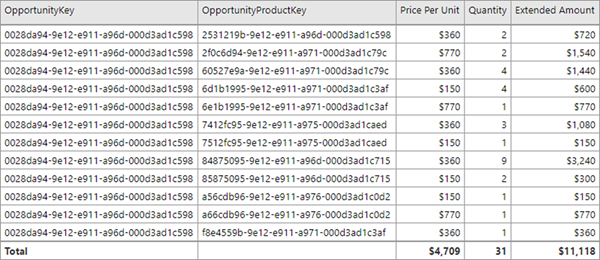I have also added three Calculated Columns and one Measure to the Opportunity Products table in Power BI.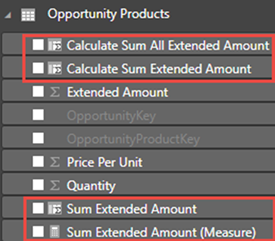These Calculated Columns and the Measure each calculate the sum of the Extended Amount. However, different values are calculated for the Sum Extended Amount, Calculate Sum Extended Amount and Calculate Sum All Extended Amount columns. These differences are because of differences in context; i.e. Row Context versus Filter Context. The differences are related to the absence or presence of the CALCULATE function and the absence or presence of Filter parameters in the CALCULATE function. They are also related to whether the calculation is being invoked for a Calculated Column or a Measure.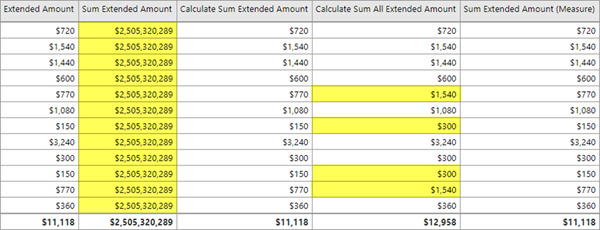Sum Extended Amount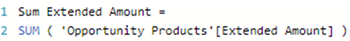This formula calculates the sum of the Extended Amount column.

Because this is a Calculated Column, there is no Filter Context and the 'Opportunity Products' [Extended Amount] expression is evaluated in a Row Context for every row in the Opportunity Products table. Because there is no Filter Context, the SUM statement aggregates the result of the 'Opportunity Products' [Extended Amount] expression for all Opportunity Products in the Opportunity Products table. This results in the same value of \$2,505,320,289 being calculated for every row.

The following formula calculates the same result because my sample data set does not include any other columns, such as a Volume Discount, that need to included in the calculation to obtain the correct result. This formula more clearly demonstrates an expression, e.g. [Price Per Unit] * [Quantity], being executed in a Row Context for a set of records before then being aggregated.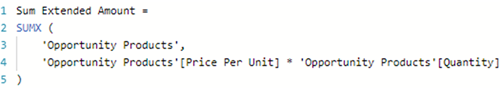Calculate Sum Extended Amount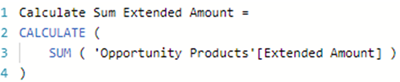This formula is the same as the Sum Extended Amount formula with the addition of CALCULATE. Here, CALCULATE is used without the addition of any Filter parameters.

When CALCULATE is used this way, it actually does apply a Filter to the expression. This causes the expression to evaluate in a Filter Context rather than a Row Context. This is known as Context Transition; i.e. a change from Row Context where no Filter Context is in effect to a Filter Context.

The Filter applied to each row by CALCULATE consists of the column values of that row. The Filter Context selects the rows with the same set of OpportunityKey, OpportunityProductKey, Price Per Unit, Quantity and Extended Amount column values as the row. Note: The OpportunityProductKey column in this example is not recognised as a Primary Key because it is not used as the parent of any one-to-many relationship in my sample data set. If it were, then the Filter Context would select the rows with the same set of OpportunityProductKey column values and would not include the other columns in the filter.
For each row, only one row is included in the Filter Context because the OpportunityProductKey makes every row unique regardless of whether or not it has been recognised as the Primary Key. The Calculate Sum Extended Amount column values are therefore the same as the Extended Amount column values for every row.

The following Measure produces the same result because behind the scenes the DAX Calculation Engine is adding CALCULATE without any additional parameters to the Measure.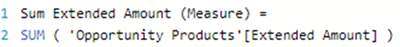Calculate Sum All Extended Amount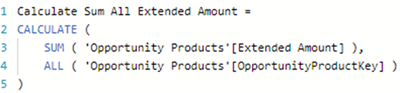Here, CALCULATE has been used with the addition of a Filter parameter that modifies the Filter Context introduced by the Context Transition. The ALL ( 'Opportunity Products'[OpportunityProductKey] ) statement removes the OpportunityProductKey column filter.

The Filter applied to each row by CALCULATE now selects the rows with the same set of OpportunityKey, Price Per Unit, Quantity and Extended Amount column values.

For those rows where this combination of column values is unique, the Calculate Sum Extended Amount column values remain the same as the Extended Amount column values because the Filter Context is selecting only one row.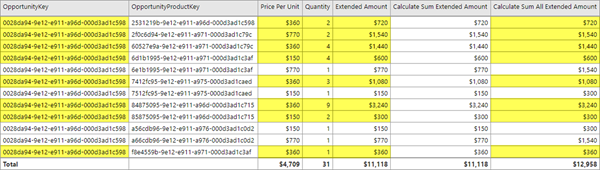However, for those rows where this combination of column values is not unique, the Calculate Sum Extended Amount column values are not the same as the Extended Amount column values because the Filter Context is selecting more than one row.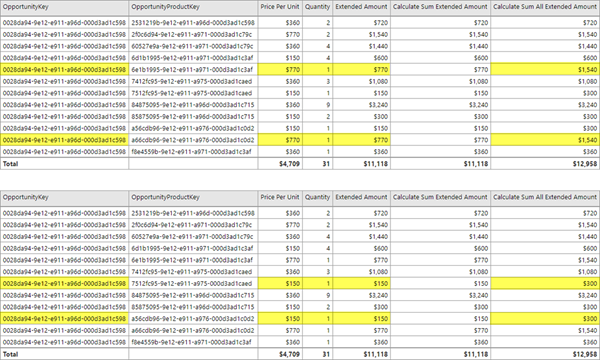If the Filter parameters in the CALCULATE statement modify the Filter Context applied by the Context Transition by removing both the OpportunityKey column and OpportunityProductKey column filters, then the results would be different again because the Filter now consists only of the Price Per Unit, Quantity and Extended Amount columns.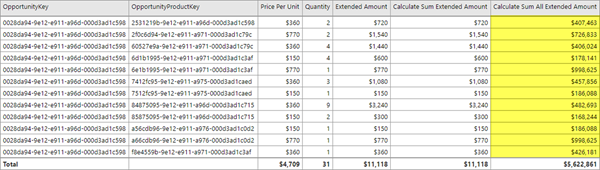Finally, if the ALL statement was changed to ALL ( 'Opportunity Products' ), then the Calculate Sum Extended Amount column value for every row would be \$2,505,320,289 because all the filters on Opportunity Products columns would be removed.